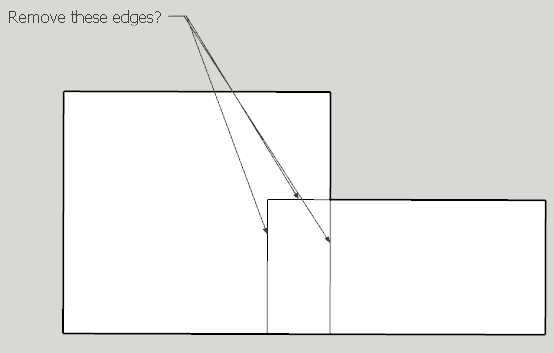# Unordered concave polygon vertices sorted

In the process of finding the union of two polygons, I got the unordered outer boundary coordinates of the union, these coordinates are disordered, I tried the method of using vector forks, it is only valid for convex polygons, but the union is a concave polygon, and it is still disordered after processing, is there any good suggestion?

``````def point_cmp(a, b, ptcenter)

return false if a.x - ptcenter.x >= 0 && b.x - ptcenter.x < 0
return true if a.x - ptcenter.x < 0 && b.x - ptcenter.x >= 0
if a.x - ptcenter.x == 0 && b.x - ptcenter.x == 0
if (a.y - ptcenter.y >= 0 || b.y - ptcenter.y >= 0)
return a.y > b.y
end
return b.y > a.y
end

det = (a.x - ptcenter.x) * (b.y - ptcenter.y) - (b.x - ptcenter.x) * (a.y - ptcenter.y)
return true if (det > 0)
return false if (det < 0)

d1 = (a.x - ptcenter.x) * (a.x - ptcenter.x) + (a.y - ptcenter.y) * (a.y - ptcenter.y)
d2 = (b.x - ptcenter.x) * (b.x - ptcenter.x) + (b.y - ptcenter.y) * (b.y - ptcenter.y)
return d1 < d2
end

def get_center_point(vPoints)
x0 = 0
y0 = 0
ptcenter = []
vPoints.length.times do|i|
x0 += vPoints[i].x
y0 += vPoints[i].y
end
ptcenter << x0/ vPoints.length.round(1)
ptcenter << y0/ vPoints.length.round(1)
return ptcenter
end

def clock_wise_sort_points(vPoints)
ptcenter = get_center_point(vPoints)
(vPoints.length-1).times do|i|
(vPoints.length-i-1).times do|j|
if point_cmp(vPoints[j],vPoints[j+1],ptcenter)
tmp = vPoints[j]
vPoints[j] = vPoints[j + 1]
vPoints[j + 1] = tmp
end
end
end
return vPoints
end
vpoints = [[0,0],[1.m,1.m],[2.m,0],[2.m,1.m],[1.m,2.m],[0,2.m]]
a = clock_wise_sort_points(vpoints)
mod = Sketchup.active_model # Open model
ent = mod.entities # All entities in model
``````

right:

my code:

This is not a solution, I’m just thinking out loud…

I guess the main issue here, there is not enough information if you just “supply” an array of the points, because there are many polygons you can create e.g.:So, You may need to determine other “restrictions” to define the “connection order” of points…

Perhaps if you explain more details about what do you mean by:

If Is these polygons are already a Faces in your model. Or In other words, do you have faces already in a model, what you want to merge and remove the edges?Then perhaps you can use this method:

or simplified version here:

Or if you share more information what you are doing and what you want to achieve…
…we may be able to give you more ideas…

2 Likes

BTW. After @jimhami42 liked my post…just came into my mind worth it to mention his method for centroid point:You might want to search for more general algorithms online: Polygon triangulation - Wikipedia

I saw the image you sent, I think the conditions I gave were insufficient. What I want to achieve is the intersection and union area of two polygons, the input multi-deformed vertex coordinate array, and the output is also the coordinate array。I looked for the Weiler-Atherton clip polygon algorithm and implemented some of the features。